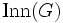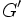# Isoclinism of groups

This is a variation of isomorphism of groups|Find other variations of isomorphism of groups |

## History

The notion of isoclinism seems to have been first introduced by Philip Hall mainly for the purpose of classifying finite p-groups, in his 1937 paper.

This page is mostly about the mappings that are used to define isoclinism. For more on the equivalence relation of being isoclinic, see isoclinic groups.

## Definition

### Short definition

An isoclinism is an isologism of groups with respect to the subvariety of abelian groups.

### Full definition

For any group$G$, let$\operatorname{Inn}(G)$ denote the inner automorphism group of$G$,$G'$ denote the derived subgroup of$G$, and$Z(G)$ denote the center of$G$.

Let$\omega_G$ denote the map from$\operatorname{Inn}(G) \times \operatorname{Inn}(G)$ to$G'$ defined by first taking the map$G \times G \to G'$ given as$(x,y) \mapsto x^{-1}y^{-1}xy$ and then observing that the map is constant on the cosets of$Z(G) \times Z(G)$.

An isoclinism of groups$G_1$ and$G_2$ is a pair$(\zeta,\varphi)$ where$\zeta$ is an isomorphism of$\operatorname{Inn}(G_1)$ with$\operatorname{Inn}(G_2)$ and$\varphi$ is an isomorphism of$G_1'$ with$G_2'$ such that$\varphi \circ \omega_{G_1} = \omega_{G_2} \circ (\zeta \times \zeta)$. Explicitly, this means that for any$x,y \in \operatorname{Inn}(G_1)$, we have the following:$\varphi(\omega_{G_1}(x,y)) = \omega_{G_2}(\zeta(x),\zeta(y))$

Pictorially, the following diagram must commute:$\begin{array}{ccc} \operatorname{Inn}(G_1) \times \operatorname{Inn}(G_1) & \stackrel{\zeta \times \zeta}{\to} & \operatorname{Inn}(G_2) \times \operatorname{Inn}(G_2) \\ \downarrow^{\omega_{G_1}} & & \downarrow^{\omega_{G_2}}\\ G_1' & \stackrel{\varphi}{\to} & G_2'\\ \end{array}$

Two groups are said to be isoclinic groups if there is an isoclinism between them.

### Definition in terms of homoclinism

An isoclinism is an invertible homoclinism of groups, i.e., a homoclinism for which both the component homomorphisms are isomorphisms. Equivalently, it is an isomorphism in the category of groups with homoclinisms.